# How Many Paths from A to B?

A popular kind of question in combinatorics is to count the number of paths between two points in a grid (following simple constraints). This can be done by very different methods at different levels. We’ll look at several problems of this type, starting with the simplest.

## Paths in a small square

We’ll start with a very simple problem; this comes from Sarah in 1999:

Finding Pathways

How do you find how many pathways there are on a square when there are three lines going across each way; from point A to point B (from top to bottom)?

The problem as stated is a little vague as to where A and B are, and what the rules are. I answered, first suggesting the usual meaning:

Hi, Sarah.

I'll fill in some details you left out, and assume that you are given a picture like this:

A
o----o----o
|    |    |
|    |    |
o----o----o
|    |    |
|    |    |
o----o----o
B

and you want to know how many ways there are to get from A to B, always going left to right or top to bottom.

I’ll show another interpretation later; but every problem in this post will follow this rule of going only in one direction on each set of lines, and will have the start and end at opposite points.

### Method 1: make an orderly list

We will be looking for efficient ways to count paths in larger grids; but this one is small enough that it makes sense just to list all possible paths, and doing so is a good way to better understand the meaning of the problem, which was my goal.

Let's number the points to make it easier to discuss:

A
1----2----3
|    |    |
|    |    |
4----5----6
|    |    |
|    |    |
7----8----9
B

Starting at A=1, you have two choices, 2 or 4, so the possible paths so far are:

1-2
1-4

From 2, there are two choices, 3 or 5; from 4, there are two choices, 5 or 7:

1-2-3
1-2-5
1-4-5
1-4-7

From 3 or 7, there are no more choices; you're forced to go one way. From 5, you have two choices. So here are all the possible paths:

1-2-3-6-9
1-2-5-6-9
1-2-5-8-9
1-4-5-6-9
1-4-5-8-9
1-4-7-8-9

So we have a total of 6 paths (in red, from green A to red B):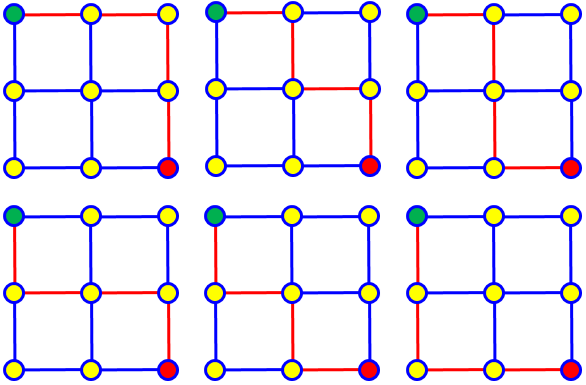If I got the constraints wrong, and you need all possible paths that don't cross, or something like that, then you can use the same basic idea to solve the real problem.

Here are all the additional paths if we remove the one-way restriction, but don’t allow a path to cross itself: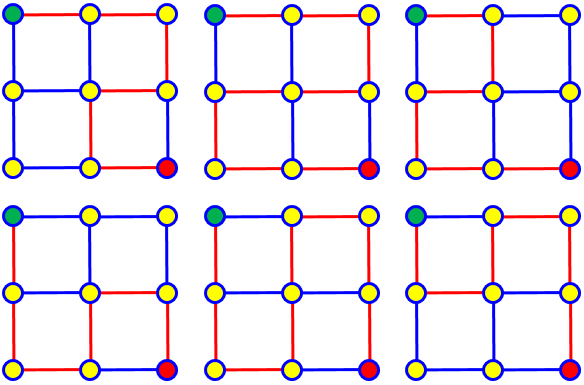I didn’t honestly expect such a rule, but wanted to make it clear that the rules were not self-evident.

### Method 2: label points with counts

Now, what if we don’t want to list the paths (as we will not, in larger problems to come)?

Another method that can be useful in problems like this is to write at each point the number of ways to get to it. If there are two places from which you can get to the same point, this number will be the sum of the number of ways to get to each of those points. Here's what it looks like for this problem:

A
1----1----1
|    |    |
|    |    |
1----2----3
|    |    |
|    |    |
1----3----6
B

Can you follow that? The 3 at what I called point 8 is the sum of the 1 at point 7 and the 2 at point 5, for example.

That is, $$1+2=3$$ here: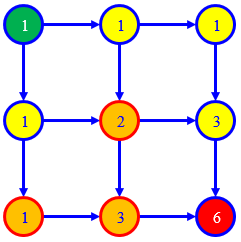In the next answer, we’ll see another, more “mathematical”, way to calculate this answer of 6.

## Paths in a larger rectangle: count ways to arrange letters

The next question is from Doug in 1996:

Number of Ways to Move

I have a group of squares which together form a larger square, i.e. a square that is 8 squares by 8 squares.

In how many ways can you travel from the upper left corner of the large square to the lower right corner by only going down or to the right?

Also, in how many ways can you do the same thing with a square that is N x N, where N is any whole number? Thanks in advance.

This is the same question, with the rules stated and a larger grid: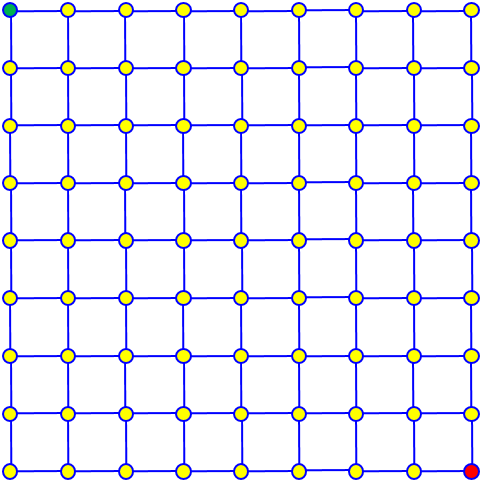In order to solve this problem, you need to start with a simple problem and then work your way to a harder one.

Let's start with a 2 by 2  matrix, that is, a square made up of 4 unit squares.  Now to get from  the upper left square to the bottom right is going to require one move  to the right and one move down.  The question is, "How many ways can I  do that?"

Let us represent a move to the right by x and a move down by  y.  Then there are two ways to complete the process: either xy or yx.

What about a 3 by 3 arrangement?  You will need two horizontal moves and  two vertical moves, that is, you need 2 x's and 2 y's.  Let's list the  number of ways you could complete the problem:

xxyy  xyxy  xyyx  yxyx  yxxy  yyxx   - a total of six ways.

Here is our practice grid: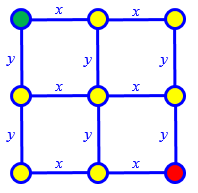The first path listed, xxyy, corresponds to the path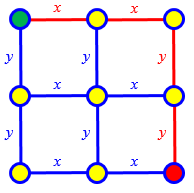Observe that what we’ve done so far is to find a useful notation, namely lists of letters representing directions, rather than my lists of points. This can be described as making an equivalent model of the problem – and that model happens to be one that is familiar to many people.

Now this problem is very similar to the one where you arrange letters in a word and have repeating letters.  For the 3 by 3 arrangement you have a  total of 4 letters (2 x's and 2 y's) but each of these is repeated, so that the number of ways they can be arranged is 4!/(2!2!) = 6  where the "!" stands for factorial.

In a 4 by 4 arrangement, the number of  possible moves would be 6!/(3!3!) = 20.

In the 8 by 8 arrangement (a  checkerboard) you asked about, the number of moves would be 14!(7!7!) = 3432.  In general, for an n x n arrangement there would be  [(n-1)+ (n-1)]!/[(n-1)!(n-1)!].  This can be simplified a bit to give  [2n-2]!/[(n-1)!]^2.

There are several ways to work out that formula, if you haven’t just memorized it. The way I think of it, for the 2×2 square, we will have 4 letters, 2 of which will be x; so we have to choose 2 positions of the 4 to be x. This is $${4\choose 2}=\frac{4!}{2!2!}=\frac{24}{4}=6$$

For the n×n case, we need to choose n positions out of $$2n$$, so the number of paths is $${{2n}\choose n}=\frac{(2n)!}{n!n!}$$

For our 8×8 grid, therefore, the number of paths is $${{16}\choose 8}=\frac{(16)!}{8!8!}=12,870$$ We wouldn’t want to list those – or even label all 81 points in the grid!

For an m×n rectangle, we need to choose m positions out of $$m+n$$, so the number of paths is $${{m+n}\choose m}=\frac{(m+n)!}{m!n!}$$

## Overlapping rectangles: find the bottlenecks

The next question is from Tracy in 2002, with a more interesting grid:

Counting Possible Paths

How do I find the number of pathways from A to B?  I know the answer is supposed to be 200, but I can't figure it out.  Please help!  What is the formula and how do you use it?

A .____.____.____.
|    |    |    |
|    |    |    |
.____.____.____.
|    |    |    |
|    |    |    |
.____.____.____.____.____.
|    |    |    |    |    |
|    |    |    |    |    |
.____.____.____.____.____.
|    |    |    |
|    |    |    |
.____.____.____.
|    |    |    |
|    |    |    |
.____.____.____.  B

Here is the grid: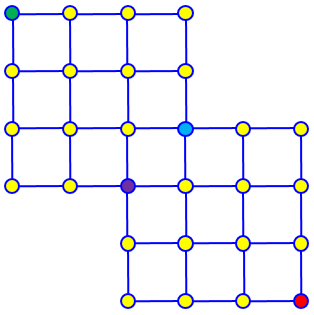The two marked points are a bottleneck, a narrow place that all paths go through.

Two of us answered with different methods.

### Method 1: combinations, two cases

Doctor Anthony wrote first:

Let's add a couple of extra points, C and D:

A .____.____.____.
|    |    |    |
|    |    |    |
.____.____.____.
|    |    |    |
|    |    |    |
.____.____.____.C___.____.
|    |    |    |    |    |
|    |    |    |    |    |
.____.____.____.____.____.
D    |    |    |
|    |    |    |
.____.____.____.
|    |    |    |
|    |    |    |
.____.____.____. B

Let H represent a horizontal step and V a vertical step.  To go from A to C requires 3 H's and 2 V's.  That is 5 steps, 3 alike of one kind and 2 alike of a second kind.

5!
Number of paths A to C = ----  = 10
3!2!

Similarly, the number of paths from A to D = 10.  Also,

5!
Number of paths from C to B =  ------ = 10
2!3!

Similarly, the number of paths from D to B = 10.

In effect, he has split the grid at points C and D, so we have two separate problems:

Paths through C: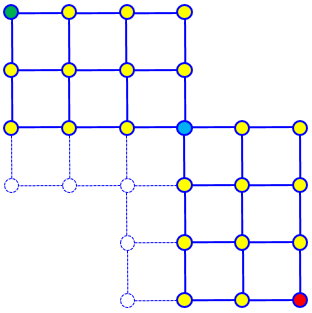Paths through D: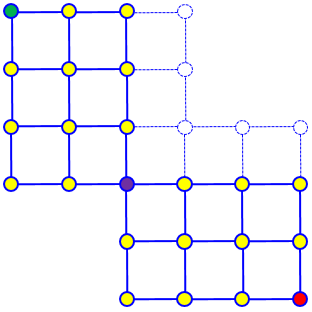Within each part of each case, he is thinking of arranging the letters in a word, either HHHVV or HHVVV.

The number of paths in each case is $${5\choose 2}\times{5\choose 3} = 10\times 10 = 100$$

Since every path has to go through C or D,

paths from A to C to B = 10 x 10  = 100

"        A  " D  " B = 10 x 10  = 100
---
total = 200

This divide-and-conquer approach allows us to use the combinations method four times. But we’ll see that this is still a relatively easy problem of its type, due to symmetry.

### Method 2: label points, using symmetry

I had been writing an answer at the same time. Since we didn’t know what level of knowledge Tracy had, I suggested the vertex-labeling method, in addition to clarifying the rules:

Hi, Tracy.

I'm assuming you are only allowed to go down and right, not to loop around.

One nice way to approach this is to label each vertex with the number of ways to get there from A. A itself has a 1, because you start there; the two next to it each have 1, because you get there only by taking that one step. Any other vertex has the sum of the numbers above and to the left, since it can be reached from either direction, and each contributes its own number of paths. Here's what we get for the left half:

A 1____1____1____1
|    |    |    |
|    |    |    |
1____2____3____4
|    |    |    |
|    |    |    |
1____3____6___10____.____.
|    |    |    |D   |    |
|    |    |    |    |    |
1____4___10____.____.____.
|C   |    |    |
|    |    |    |
.____.____.____.
|    |    |    |
|    |    |    |
.____.____.____.  B

We could continue; but because of symmetry, you can see that there are likewise 10 ways to get from either of the middle vertices to B. That gives 10*10 ways to get from A to B via C, and 10*10 ways via D. In all: 200 ways!

We can see that the number of ways to get from C to B is the same as the number of ways to get from B to C, which is identical to getting from A to D (if you turn the grid around); that is the symmetry we are using.

You may recognize the numbers we got as part of Pascal's Triangle. That gives another way to solve this without having to do our own counting. Or, you can use combinations: for example, to get to point C, you need to take 5 paths, of which 2 are right and 3 are down. The number of ways to choose 2 of the 5 (in order) to go right is C(5,2) = 10.

Some people call this the Pascal’s Triangle method, because we are adding numbers in the same way we do there. And the use of combinations (binomial coefficients) to calculate the same triangle ties this right back to what Doctor Anthony did. Our two approaches are ultimately two ways to do the same thing.

These methods are harder once you get past the bottleneck, or if you had a more complicated set of paths; the symmetry I pointed out is then a big help. But the labeling method works for any such problem.

I didn’t actually complete my method past the “bottleneck” of points C and D; I’ll do that in a new problem below.

## A little harder, now

Tracy quickly wrote back, wisely wondering what to do when there’s no symmetry:

What if it isn't pretty and symmetrical like that one?

A .____.____.____.
|    |    |    |
|    |    |    |
.____.____.____.____.____.
|    |    |    |    |    |
|    |    |    |    |    |
.____.____.____.____.____.
|    |    |    |    |    |
|    |    |    |    |    |
.____.____.____.____.____.
|    |    |
|    |    |
.____.____.B

What if it's like this one?

### Combinations

Again, we both responded. First, Doctor Anthony:

A .____.____.____.
|    |    |    |
|    |    |    |
.____.____.____.C___.F___.
|    |    |    |    |    |
|    |    |    |    |    |
.____.____.____.D___.G___.
|    |    |    |    |    |
|    |    |    |    |    |
.____.____.____.E___.H___.
|    |    |
|    |    |
.____.____.B

Work out the paths

A->C->F->B
A->D->G->B
A->E->B

and add the three results.

This takes more thought, as the link between the two parts is wider. In effect, he is cutting the grid at the two lines and one point I colored in red above.

Here is how he is dividing the grid: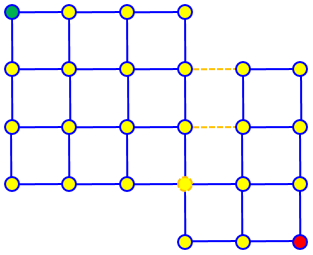Here are the three cases: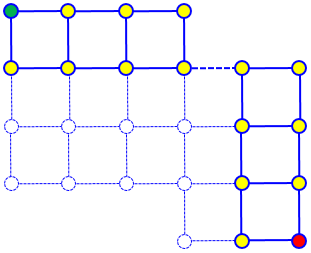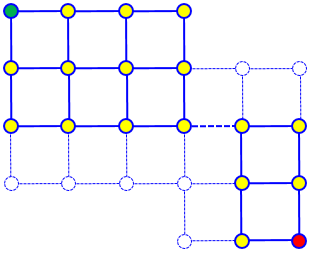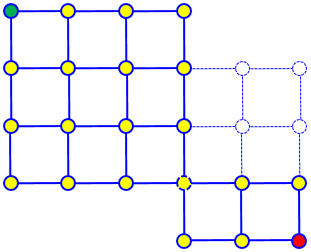The result is $${4\choose 3}\times{4\choose 1} + {5\choose 3}\times{3\choose 1} + {6\choose 3}\times{3\choose 2} = 4\times 4+10\times 3+20\times 3 = 16+30+60 = 106$$

### Label points

I wrote the next day:

Hi, Tracy.

Just to supplement Dr. Anthony's answer, here's the work for my labeling method:

A 1____1____1____1
|    |    |    |
|    |    |    |
1____2____3____4____4____4
|    |    |    |    |    |
|    |    |    |    |    |
1____3____6___10___14___18
|    |    |    |    |    |
|    |    |    |    |    |
1____4___10___20___34___52
|    |    |
|    |    |
20___54___106 B

That takes very little thinking, and isn't much slower for small problems like this.

Now, in the symmetrical grid I handled the second rectangle backward, counting from B back to C and D. Could I do that here?

Incidentally, the method equivalent to Dr. Anthony's suggestion in this case is to treat the three vertices in the middle (where the two rectangles meet) as a bottleneck, and calculate for each of those both how many ways there are to get there from A, and how many ways there are to get from there to B; then multiply for each and add them up:

From A:             To B: (adding numbers to right and below)

A 1____1____1____1
|    |    |    |
|    |    |    |
1____2____3____4  4____4____1  (Note that we don't include any
|    |    |    |       |    |   line in both parts, to avoid
|    |    |    |       |    |   counting any paths twice)
1____3____6___10  3____3____1
|    |    |    |       |    |
|    |    |    |       |    |
1____4___10___20  3____2____1
|    |    |
|    |    |
1____1____1 B

Total ways:

4*4 + 10*3 + 20*3 = 16 + 30 + 60 = 106

Dr. Anthony's calculations give these same numbers without actually counting. Obviously you have to be pretty careful!

## A bigger bottleneck: summing combinations

A similar question came from Jeremy, later in 2002:

Counting Paths With Factorials

I want to solve a problem using factorials instead of labelling using Pascal's Triangle.

The question:

How many possible ways are there to get from point A to point B.

A  _______________
|   |   |   |   |
|___|___|___|___|
|   |   |   |   |
|___|___|___|___|___________
|   |   |   |   |   |   |   |
|___|___|___|___|___|___|___|
|   |   |   |   |   |   |   |
|___|___|___|___|___|___|___|
|   |   |   |   |   |
|___|___|___|___|___|
B

I've solved it using Pascal's Triangle (the number of possible routes moving from point A to point B is 690). I want to solve it with factorials, i.e. x!/y!...  I think I am stuck with splitting the figures up because if you do so, there will be too many squares that share a side.

I've been told that it is possible to solve it using factorials. Thanks!

We can guess that “factorials” to him means “combinatorics” in general; and the Pascal’s Triangle method would be labeling points.

Let’s check the labeling method, since Jeremy didn’t show his work:

  1___1___1___1___1
|   |   |   |   |
1___2___3___4___5
|   |   |   |   |
1___3___6__10__15__15__15__15
|   |   |   |   |   |   |   |
1___4__10__20__35__50__65__80
|   |   |   |   |   |   |   |
1___5__15__35__70_120_185_265
|   |   |   |   |   |
15__50_120_240_425_690

Hello, Jeremy --

I'm not sure what you mean by solving this using factorials.  It can be solved with repeated use of the concept of 'n choose r', which involves factorials....

I first verified your answer by using the old Pascal's triangle method. Then I tried several different ways to come up with that same answer; most of these ways involved starting with the total number of ways to make the trip if the missing corner squares were NOT missing (C(12,5) and counting the number of paths that can't be used because they ARE missing. All of these approaches proved too awkward.

We’ll use this subtractive method later, for two simpler problems.

He ends up using a bottleneck method:

Then I finally hit upon a relatively simple solution using C(n,r).

If you analyze your figure carefully, you will see that every solution path must go through exactly one of the three points which I have labeled in the diagram below as X, Y, and Z:

A  _______________
|   |   |   |   |
|___|___|___|___|
|   |   |   |   |
|___|___|___|___|___________
|   |   |   |  X|   |   |   |
|___|___|___|___|___|___|___|
|   |   |  Y|   |   |   |   |
|___|___|___|___|___|___|___|
Z|   |   |   |   |   |
|___|___|___|___|___|
B

Because these three points are not above one another, as in that last example, we just have to “snip” at those points rather than along lines.

This fact makes counting the paths relatively easy:

The number of paths through X is the number of paths from A to X times the number of paths from X to B:

C(6,2)*C(6,3) = 15*20 = 300

The number of paths through Y is the number of paths from A to Y times the number of paths from Y to B:

C(6,3)*C(6,2) = 20*15 = 300

The number of paths through Z is the number of paths from A to Z times the number of paths from Z to B:

C(6,2)*C(6,1) = 15*6 = 90

The total number of paths is 300+300+90 = 690.

## A subtractive approach

We’ll end with this question from 2014:

Paths Walked, Worked Backwards

A beetle is standing at point A:

A -----------
|   |   |   |
-----------------
|   |   |   |
------------B

It only walks right and down, and it always stays on the lines.

How many different paths can it take to point B?

I looked for a hint in Doctor Ricky's conversation, "Possible Paths across a Rectangular Grid":
http://mathforum.org/library/drmath/view/66728.html

However, I'm still confused on how to remove paths that are not covered in the grid. I'm not sure how to solve this problem without explicitly counting all the routes.

The answer from Doctor Ricky is one of several we have omitted; it involved a simple rectangle, and showed the approach of counting combinations of horizontal and vertical moves. I answered, referring to the long discussion above:

Hi, Anuj.

You should choose the way that exercises whichever kind of skill you are meant to be learning!

Here, you can see a couple different approaches to a problem much like yours:

Counting Possible Paths
http://mathforum.org/library/drmath/view/60784.html

One way involves combinations, adding and multiplying to stitch parts of the grid together. The other involves nothing more than a quick way to count. (The second example is particularly like yours.)

Now, you seem to be suggesting a third way, starting from a larger rectangle and subtracting, rather than adding and multiplying smaller sets of paths. That's probably not a bad idea for this problem.

I don’t know whether Anuj invented this idea alone (perhaps because the grid is so close to a larger rectangle), or it was suggested. But it was a very good idea.

Let me see how I'd do it:

A---+---+---+   X
|   |   |   |
+---+---+---+---+
|   |   |   |
Y   +---+---+---B

You can find the number of ways to get from A to B in the complete grid below:

A---+---+---+---X
|   |   |   |   |
+---+---+---+---+
|   |   |   |   |
Y---+---+---+---B

Then subtract out the (very small!) number of ways to get from A to B via X or via Y.

Yes, that works nicely; and it's a lot easier than Dr. Anthony's additive method. (It works well on the second problem on the page above, too.)

I'd suggest trying two or three of these methods, as a check on your answer.

The total number of paths including X and Y is $${6\choose 4}=15$$ There is exactly one way each through X and through Y, so the answer is $$15-1-1 = 13$$. We get the same answer by labeling:

   1---1---1---1
|   |   |   |
1---2---3---4---4
|   |   |   |
2---5---9---13

Let’s try Tracy’s second problem: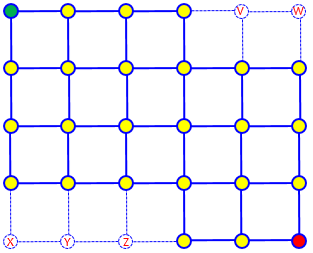The total number of paths in the rectangle is $${9\choose 5}=126$$.

The number of paths through V is $${5\choose 1}=5$$; all those that go through W are included. The number of paths through Z is $${6\choose 2}=15$$; all those that go through X or Y are included. So we have to subtract $$5+15=20$$ from 126, giving a net of $$126-20=106$$. That’s what we got before. There’s some subtle thinking, but not as much as in the additive method.

### 1 thought on “How Many Paths from A to B?”

This site uses Akismet to reduce spam. Learn how your comment data is processed.